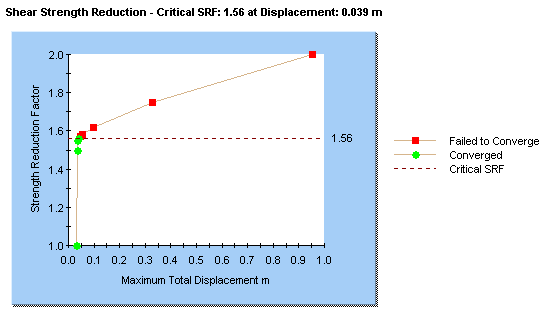Join one of our upcoming courses taking place around the world! Explore now

# Graph SSR Results

If you have carried out a Shear Strength Reduction (SSR) analysis, then you can plot the Shear Strength Reduction Factor versus the Maximum Displacement, by selecting the Graph Shear Strength Reduction option from the Graph menu.

A typical graph looks similar to the following figure.

Shear Strength Reduction graphThis graph represents the essential results of the SSR Analysis:

• Each data point represents the Shear Strength Reduction Factor versus the Maximum Displacement, for one iteration of the SSR analysis. The Maximum Displacement is the Maximum value of Total Displacement which occurred at any point in the model, for the stress analysis which was computed using that value of Strength Reduction Factor.
• Data points displayed in green represent analyses for which the stress analysis results achieved convergence within the specified tolerance for the analysis. (The tolerance is the Tolerance (stress analysis) value under the Strength Reduction tab of the Project Settings dialog).
• Data points displayed in red represent analyses for which the stress analysis did NOT achieve convergence within the specified tolerance (i.e. the model was unstable).

## Critical Strength Reduction Factor

The Critical Strength Reduction Factor (Critical SRF) is the maximum value of SRF for which the model remains stable (i.e. the analysis converges). This is the uppermost green data point on the graph, and is marked by a horizontal dotted line on the graph.